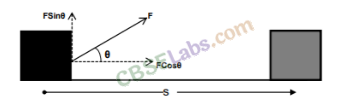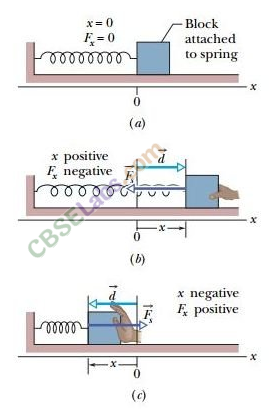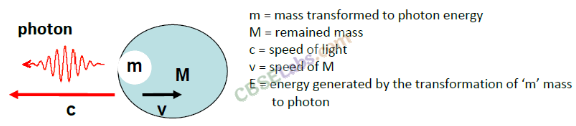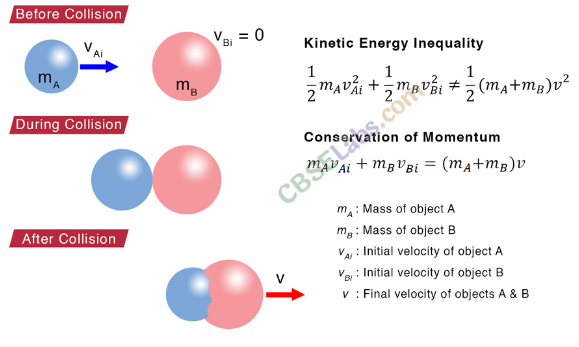# CBSE Class 11 Physics Chapter 6 Revision Notes

Chapter 6: Work, Energy and Power Revision Notes

• When a force is applied to the body, it causes the body to move a certain distance in the direction of the applied force, work is said to be done.
• It is calculated as the product of the force and the distance traveled in the force’s direction, i.e. W = F-S.
• If an object undergoes displacement ‘S’ along a straight line while being acted on by a force F, it forms an angle of 0 with S.
• The product of the component of force in the direction of displacement and the magnitude of displacement is the work done W by the agent.Source

• If a spring is stretched or compressed by a small distance from its unstretched configuration, it will exert a force on the block equal to F = -kx, where x is the spring’s compression or elongation, and k is a constant called the spring constant, whose value is inversely proportional to the spring’s unstretched length and the nature of the spring’s material.
• The negative sign indicates that the spring force is in the opposite direction of x, the free-end displacement.Source

• Energy: A body’s energy is its ability to perform tasks. Anything that has the ability to work is said to have energy. The unit of energy is the same as the unit of work, the Joule.

• Kinetic and potential energy are the two types of mechanical energy.

• Kinetic energy is the energy possessed by a body as a result of its motion.

• The kinetic energy of an object with mass m and velocity v is given by:

K.E. or K = 1/2 mv2

• Potential energy is the energy possessed by a body as a result of its position or condition.

• Potential energy is divided into two types: gravitational and elastic.

• The energy possessed by a body as a result of its position above the earth’s surface is known as gravitational potential energy.

It is given by (U)P.E. = mgh, where m denotes the mass of a body and g denotes the acceleration due to gravity on the earth’s surface. h is the height at which the body is lifted.

• Work must be done against the restoring elastic force when an elastic body is displaced from its equilibrium position. The work that is done is stored in the body as elastic potential energy.
• If an elastic spring is stretched (or compressed) by a distance Y from its equilibrium position, its elastic potential energy is U= 1/2 kx2, where k is the spring’s force constant.

### The Law of Energy Conservation

• The total energy of an isolated system does not change, according to the law of conservation of energy. Although energy can be converted from one form to another, an isolated system’s total energy remains constant.
• Neither the creation nor the destruction of energy are possible. Other than mechanical energy, energy can take many different forms.
• Thermal energy, electrical energy, chemical energy, visual light energy, nuclear energy, and so on are some of these forms.

Mass and energy equivalence: Mass and energy, according to Einstein, are interchangeable. That is, mass can be converted into energy and vice versa.Source

## Collision

• Collision is defined as a one-time occurrence in which two or more colliding bodies exert relatively strong forces on each other for a short period of time.
• Collisions between particles can be divided into two categories.

Elastic Collision

If both the linear momentum and the kinetic energy of the system are conserved, a collision between two particles or bodies is said to be elastic.

Collisions of atomic particles, atoms, marble balls, and billiard balls, for example.

Inelastic Collision

If the system’s linear momentum is conserved but its kinetic energy is not, the collision is said to be inelastic.

When we drop a ball of wet putty on the floor, for example, the collision between the ball and the floor is inelastic.

• If the colliding particles move along the same straight line path both before and after the collision, the collision is said to be one dimensional.
• The relative velocity of approach before collision is equal to the relative velocity of separation after collision in a one-dimensional elastic collision.Source

]]>